This site uses cookies. By continuing to browse the ConceptDraw site you are agreeing to our Use of Site Cookies.
ConceptDraw Samples | Computer and networks — Computer network diagrams

# Computer Network Diagrams

Samples of computer network diagrams (computer network architecture, topology, and design; logical, physical, cable networks; rack diagrams) are created using ConceptDraw DIAGRAM diagramming and vector drawing software enhanced with Solutions from Computer and Networks area of ConceptDraw Solution Park. ConceptDraw DIAGRAM provides export of vector graphic multipage documents into multiple file formats: vector graphics (SVG, EMF, EPS), bitmap graphics (PNG, JPEG, GIF, BMP, TIFF), web documents (HTML, PDF), PowerPoint presentations (PPT), Adobe Flash (SWF).

## Tutorials and Solutions:

Video Tutorials: ConceptDraw Solution Park

Solutions: Computer and Networks for ConceptDraw DIAGRAM

## Sample 1: Network Diagram

Network Diagram sample.

This example is created using ConceptDraw DIAGRAM diagramming and vector drawing software enhanced with Computer and Networks solution from ConceptDraw Solution Park.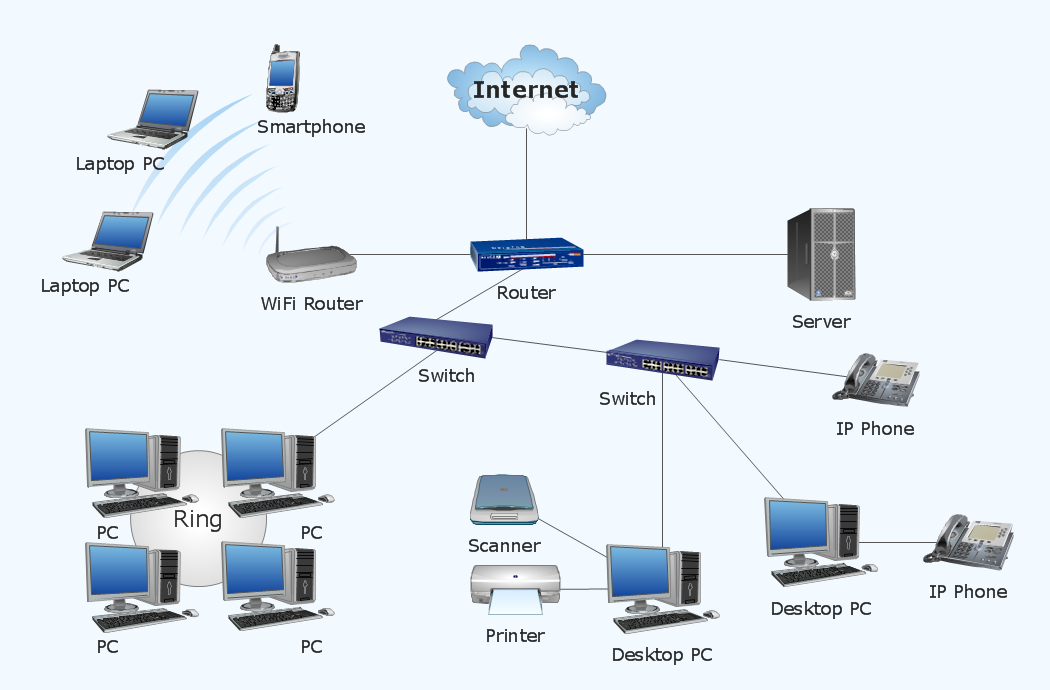## Sample 2: Network Diagram — Active Directory Structure

Network Diagram sample: Active directory structure.

This example is created using ConceptDraw DIAGRAM diagramming and vector drawing software enhanced with Computer and Networks solution from ConceptDraw Solution Park.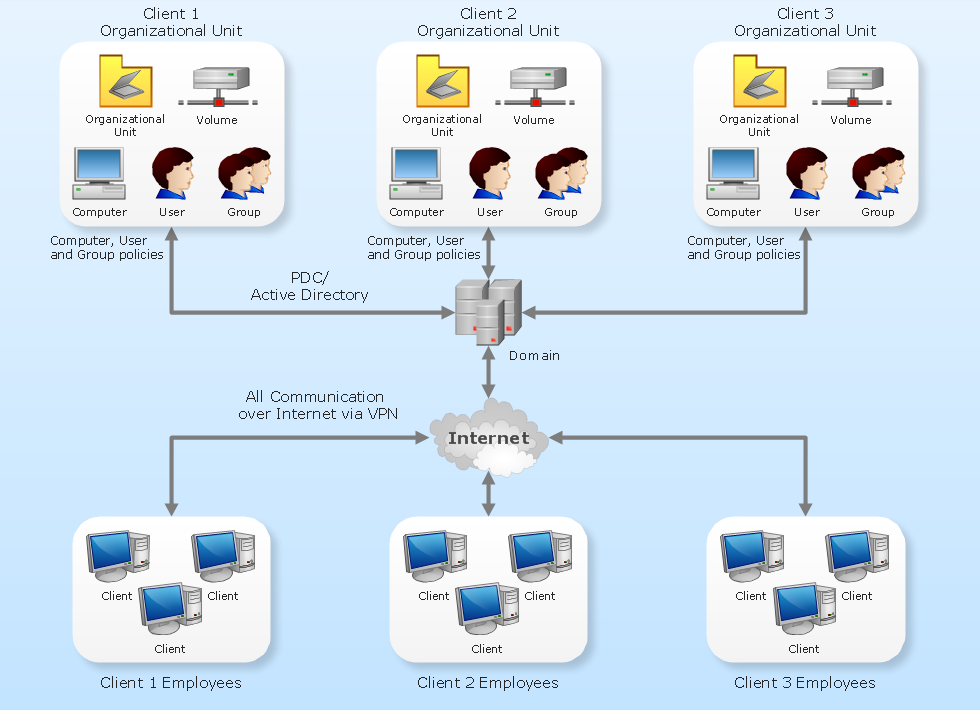## Sample 3: Rack Diagram

Rack Diagram sample.

This example is created using ConceptDraw DIAGRAM diagramming and vector drawing software enhanced with Computer and Networks solution from ConceptDraw Solution Park.## Sample 4: Cisco Network Diagram — LAN Fault Tolerance System

Cisco Network Diagram sample: LAN fault tolerance system.

This example is created using ConceptDraw DIAGRAM diagramming and vector drawing software enhanced with Computer and Networks solution from ConceptDraw Solution Park.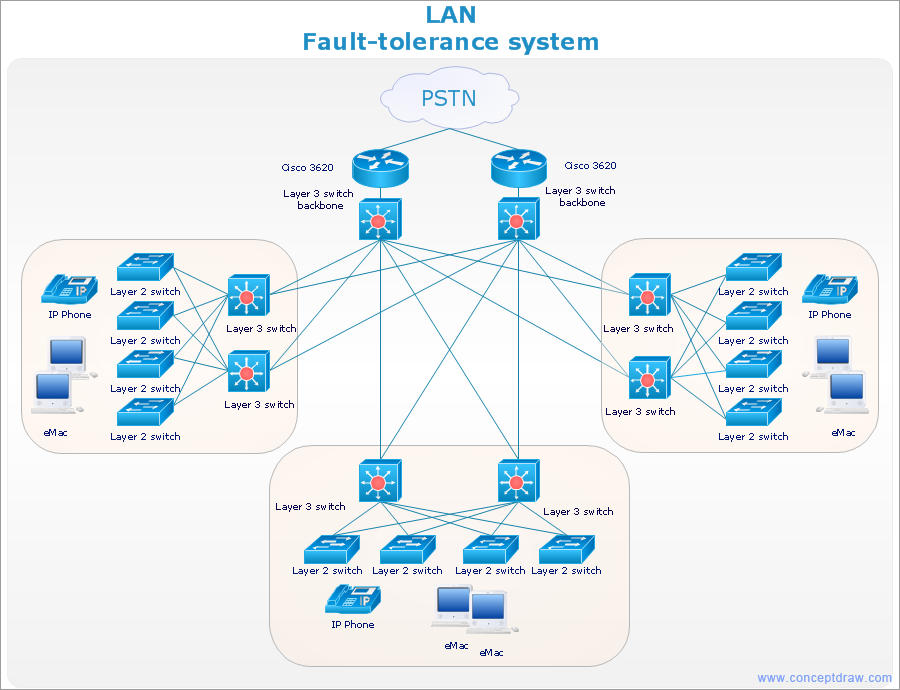## Sample 5: Network Topology Diagram — Cisco Intelligent Services Gateway (ISG)

Network Topology diagram sample: Cisco Intelligent Services Gateway (ISG).

This example is created using ConceptDraw DIAGRAM diagramming and vector drawing software enhanced with Computer and Networks solution from ConceptDraw Solution Park.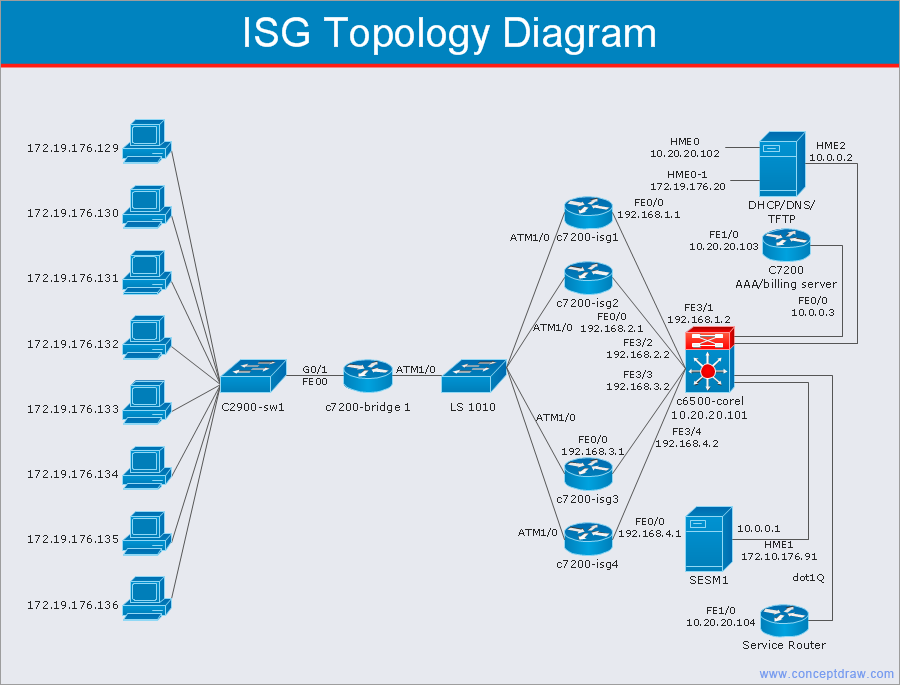## Sample 6: Network Diagram — Call Center

Network Diagram sample: Call Center.

This example is created using ConceptDraw DIAGRAM diagramming and vector drawing software enhanced with Computer and Networks solution from ConceptDraw Solution Park.## Sample 7: Network Diagram — Active Directory Domain Services

Network Diagram sample: Active directory domain services.

This example is created using ConceptDraw DIAGRAM diagramming and vector drawing software enhanced with Computer and Networks solution from ConceptDraw Solution Park.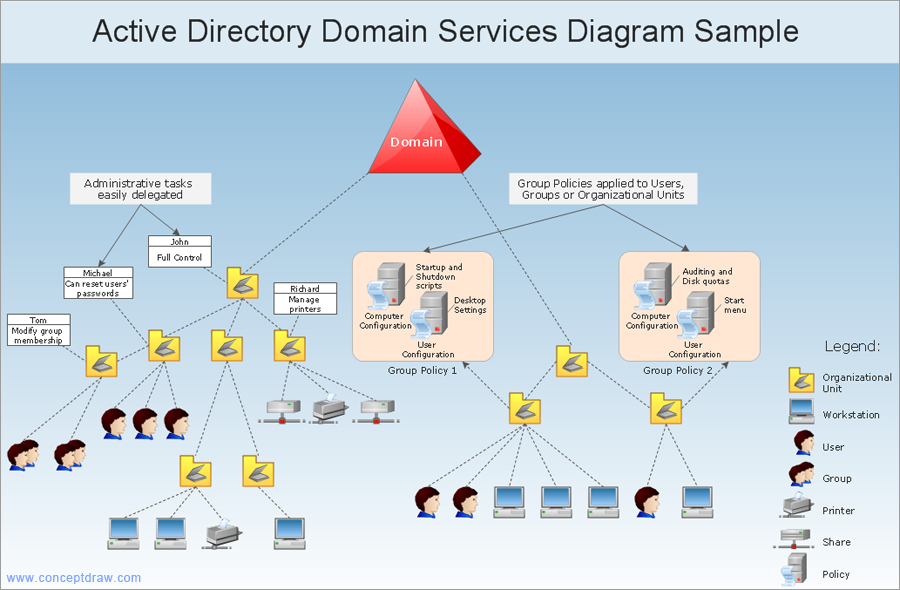## Sample 8: Cisco Network Diagram

Network Diagram sample: Cisco Network Diagram.

This example is created using ConceptDraw DIAGRAM diagramming and vector drawing software enhanced with Computer and Networks solution from ConceptDraw Solution Park.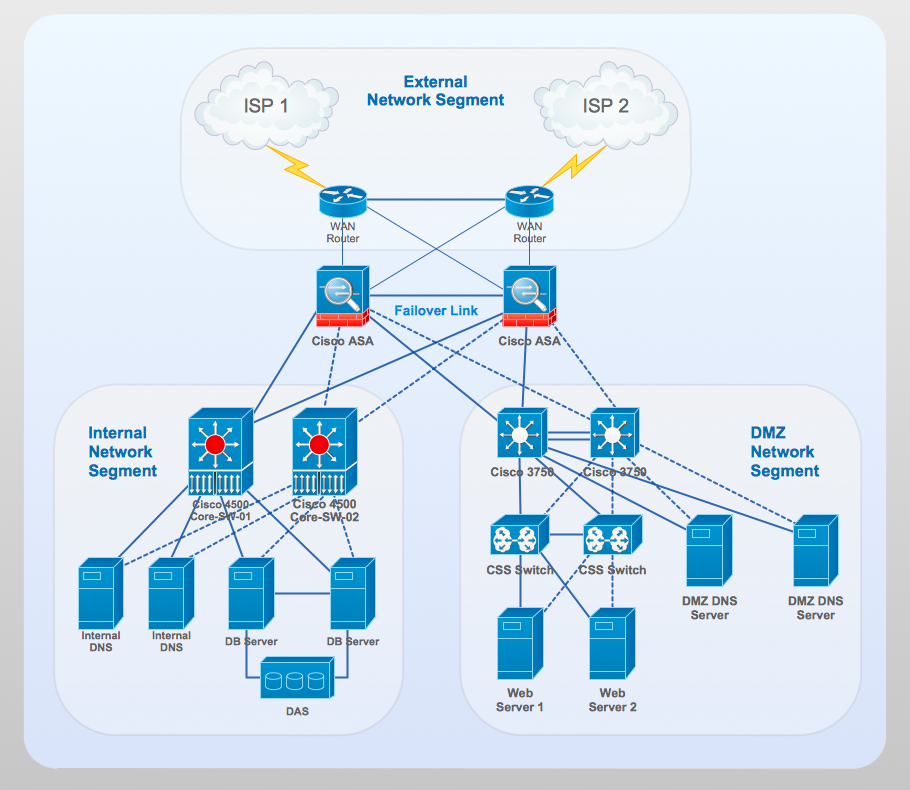## Sample 9: Typical Server Rack Diagram

Rack Diagram sample: Typical server rack.

This example is created using ConceptDraw DIAGRAM diagramming and vector drawing software enhanced with Computer and Networks solution from ConceptDraw Solution Park.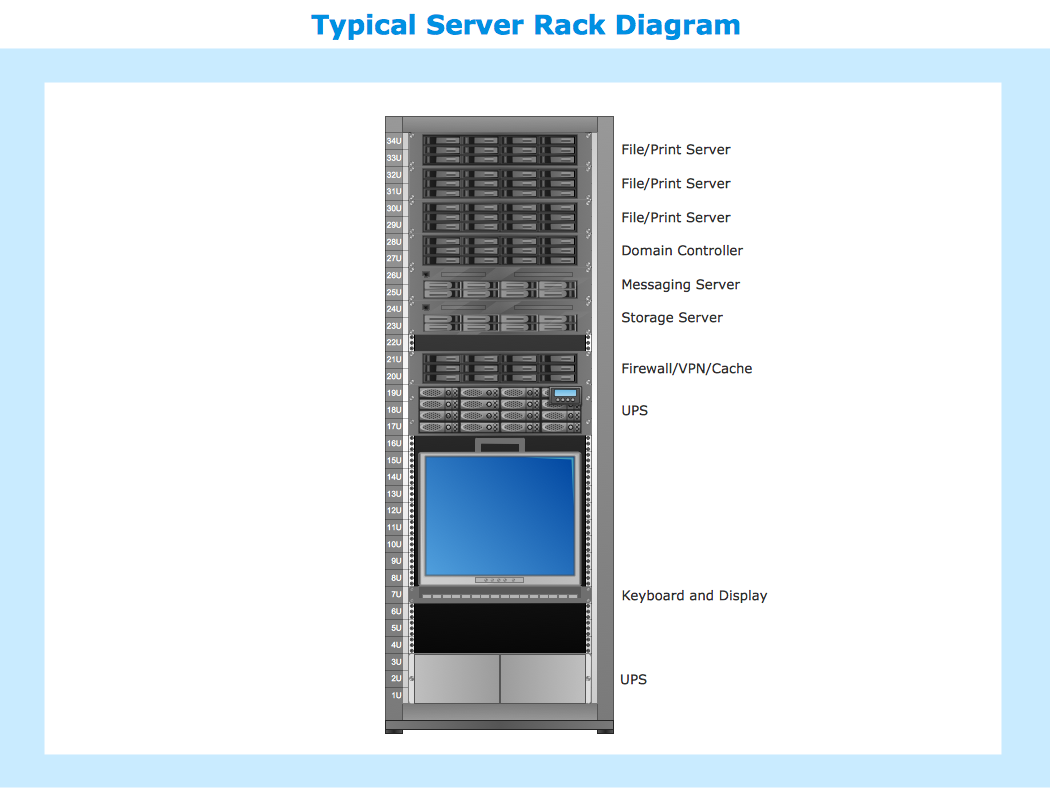## Sample 10: Network Diagram — System Design

Network Diagram sample: System design.

This example is created using ConceptDraw DIAGRAM diagramming and vector drawing software enhanced with Computer and Networks solution from ConceptDraw Solution Park.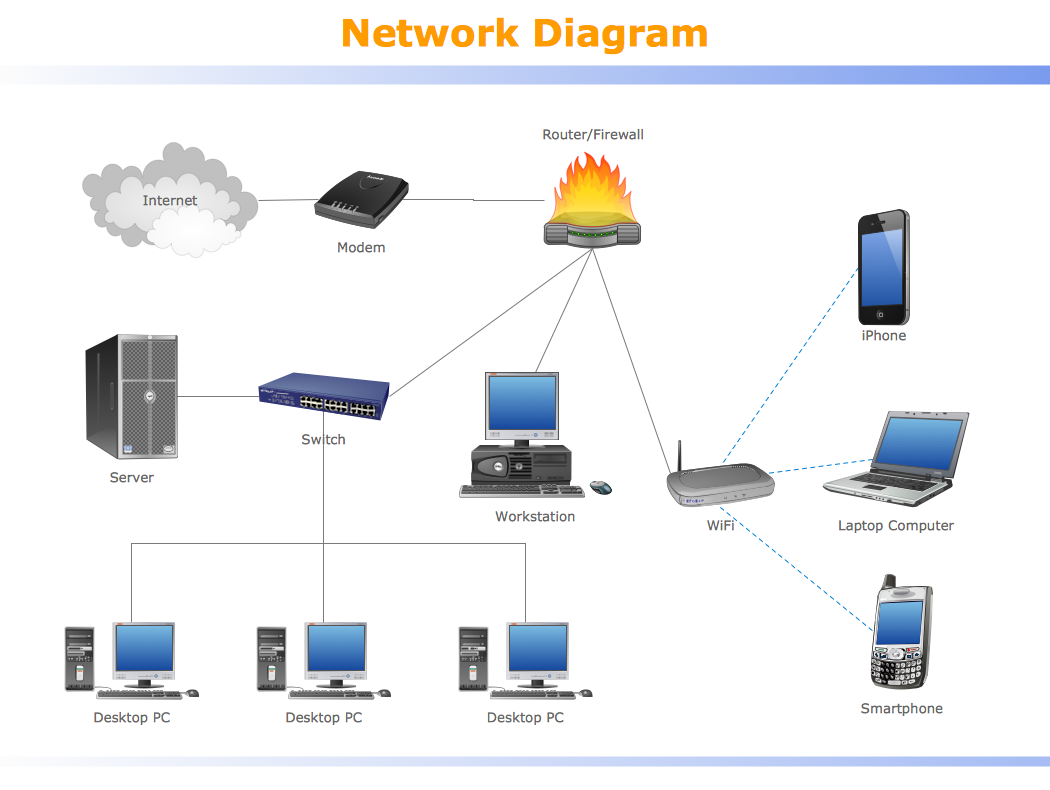## Sample 11: Network Diagram — Simple LAN

Network Diagram sample: Simple LAN.

This example is created using ConceptDraw DIAGRAM diagramming and vector drawing software enhanced with Computer and Networks solution from ConceptDraw Solution Park.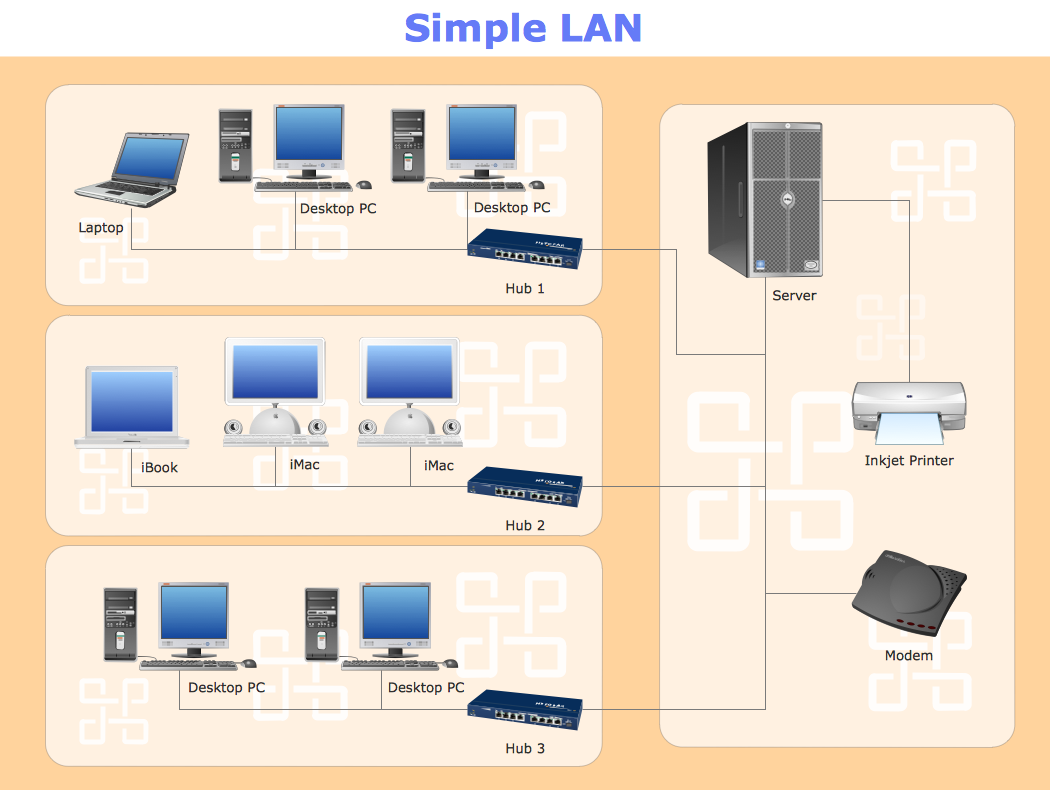## Sample 12: Cisco Network Organization Diagram

Cisco Network Organization Diagram sample.

This example is created using ConceptDraw DIAGRAM diagramming and vector drawing software enhanced with Computer and Networks solution from ConceptDraw Solution Park.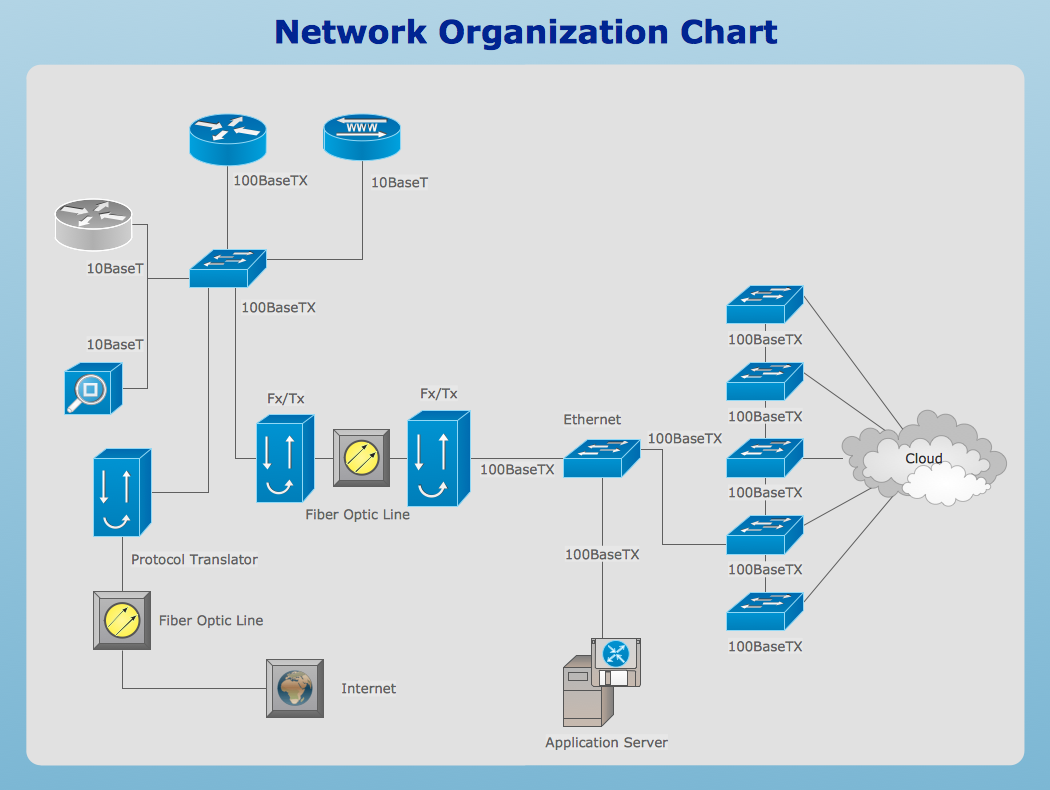## Sample 13: Network Floor Plan Layout

Network Floor Plan Layout sample.

This example is created using ConceptDraw DIAGRAM diagramming and vector drawing software enhanced with Computer and Networks solution from ConceptDraw Solution Park.## Sample 14: Network Diagram

Network Diagram sample.

This example is created using ConceptDraw DIAGRAM diagramming and vector drawing software enhanced with Computer and Networks solution from ConceptDraw Solution Park.## Sample 15: Cisco Logical Network Diagram

Cisco Logical Network Diagram sample.

This example is created using ConceptDraw DIAGRAM diagramming and vector drawing software enhanced with Computer and Networks solution from ConceptDraw Solution Park.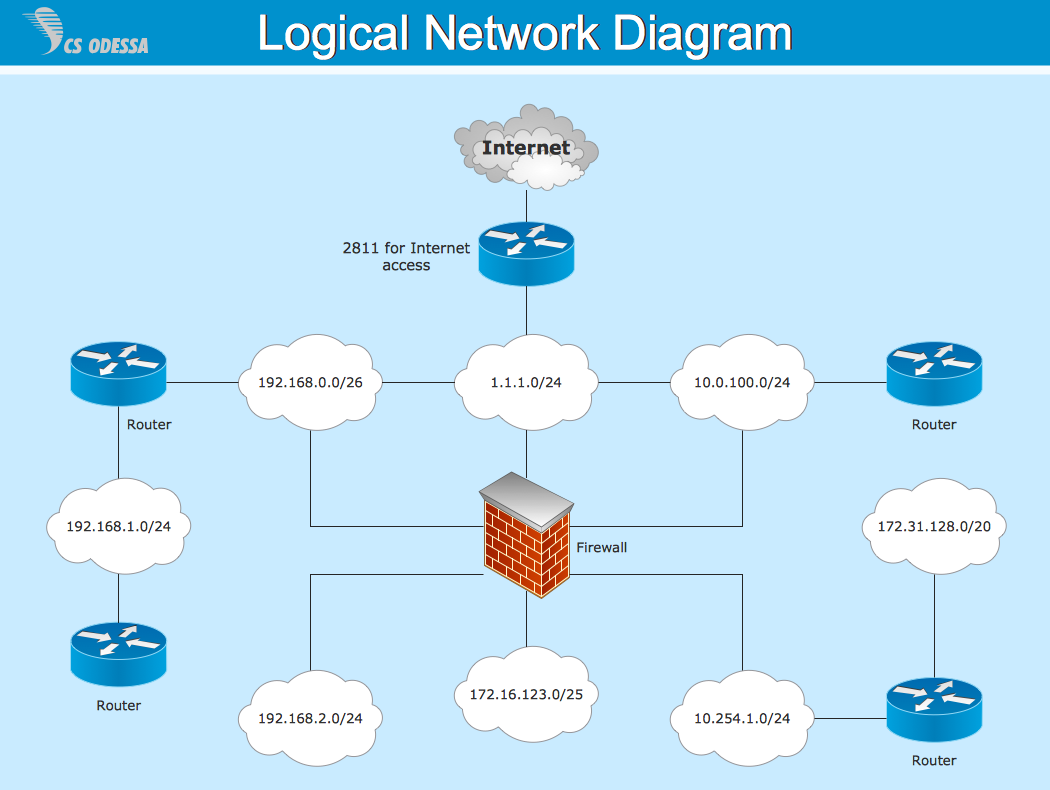## Sample 16: Hybrid Satellite & Common Carrier Networks Diagram

Network Diagram sample: Hybrid satellite & common carrier networks.

This example is created using ConceptDraw DIAGRAM diagramming and vector drawing software enhanced with Computer and Networks solution from ConceptDraw Solution Park.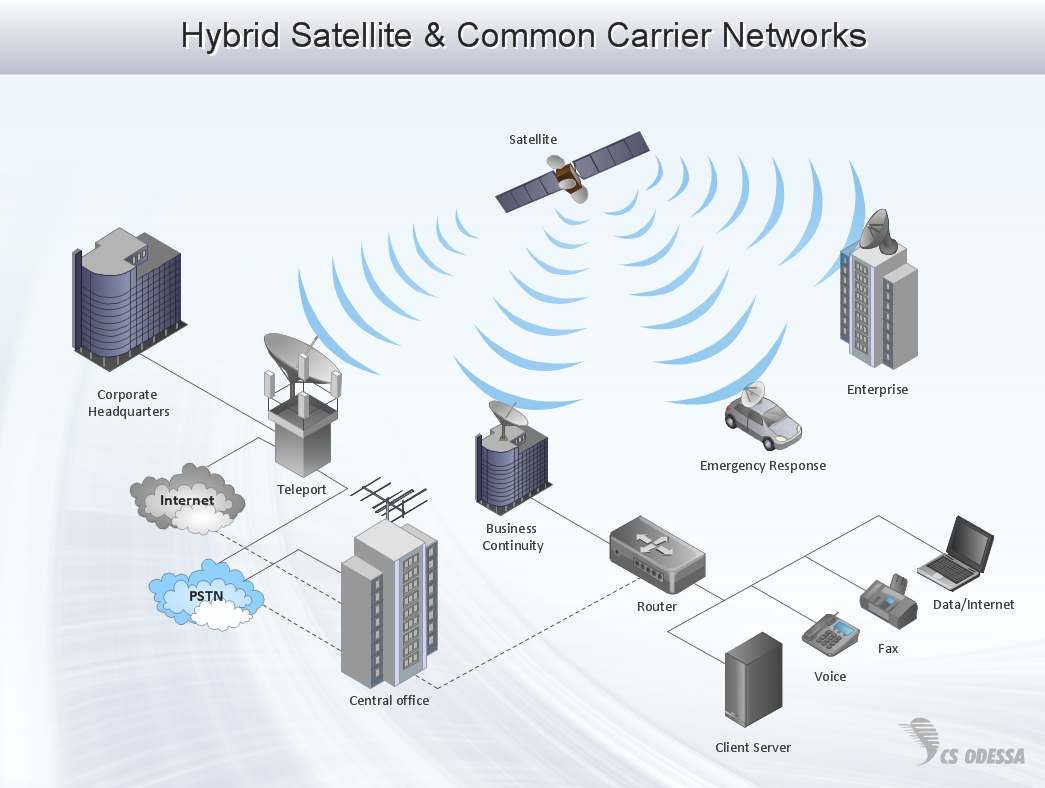## Sample 17: Crossover Cable Wiring Diagram

Network Diagram sample: Crossover Cable Wiring Diagram.

This example is created using ConceptDraw DIAGRAM diagramming and vector drawing software enhanced with Computer and Networks solution from ConceptDraw Solution Park.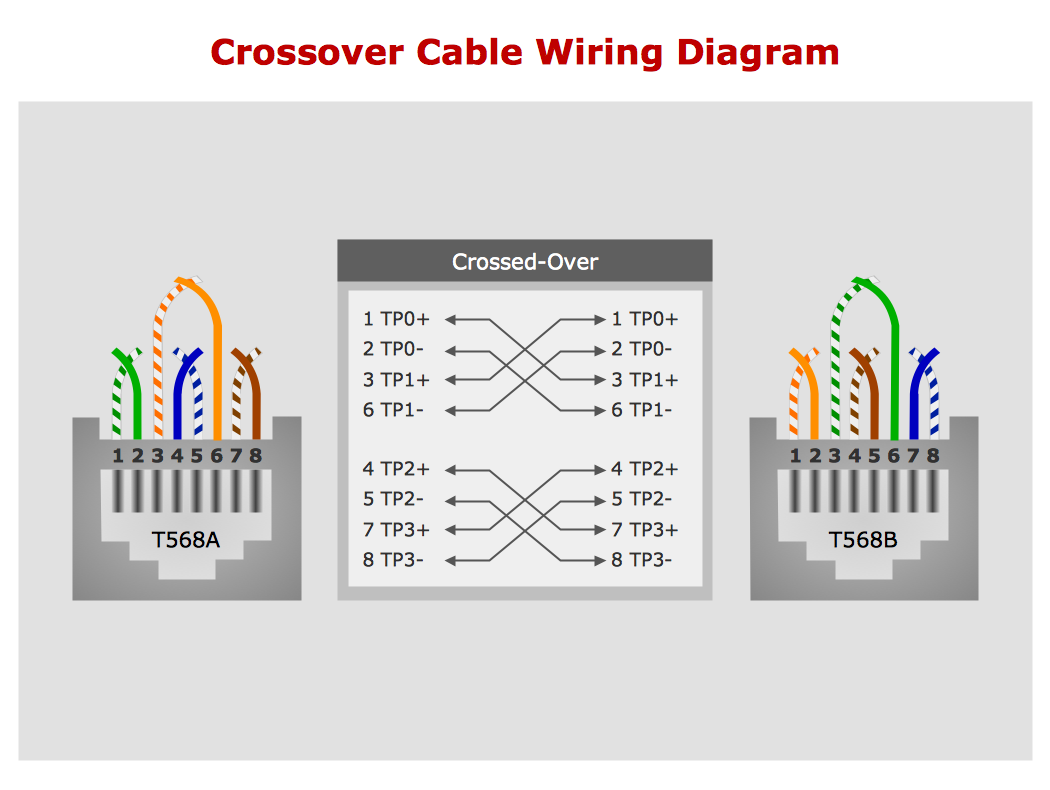## Sample 18: Network Diagram — Bus Topology Diargram

Network Diagram sample: Bus topology diargram.

This example is created using ConceptDraw DIAGRAM diagramming and vector drawing software enhanced with Computer and Networks solution from ConceptDraw Solution Park.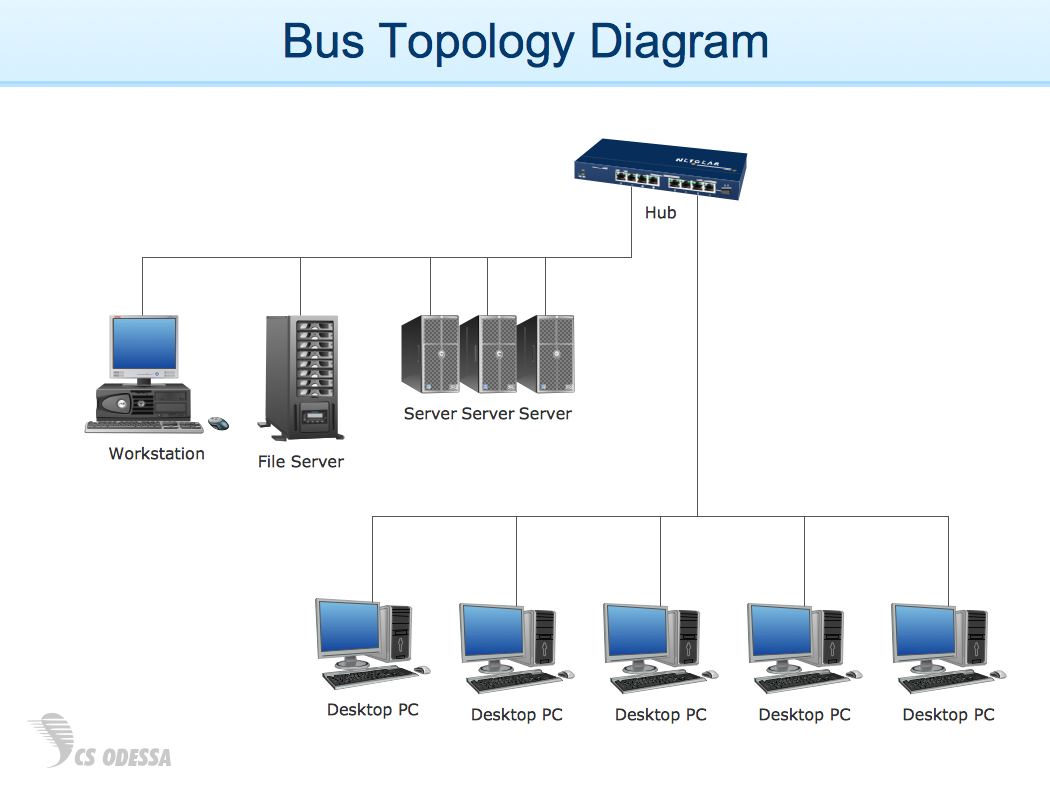## Sample 19: Network Diagram Sample

Network Diagram sample.

This example is created using ConceptDraw DIAGRAM diagramming and vector drawing software enhanced with Computer and Networks solution from ConceptDraw Solution Park.## Sample 20: Rack Diagram — Application Server Network

Rack Diagram sample: Application server network.

This example is created using ConceptDraw DIAGRAM diagramming and vector drawing software enhanced with Computer and Networks solution from ConceptDraw Solution Park.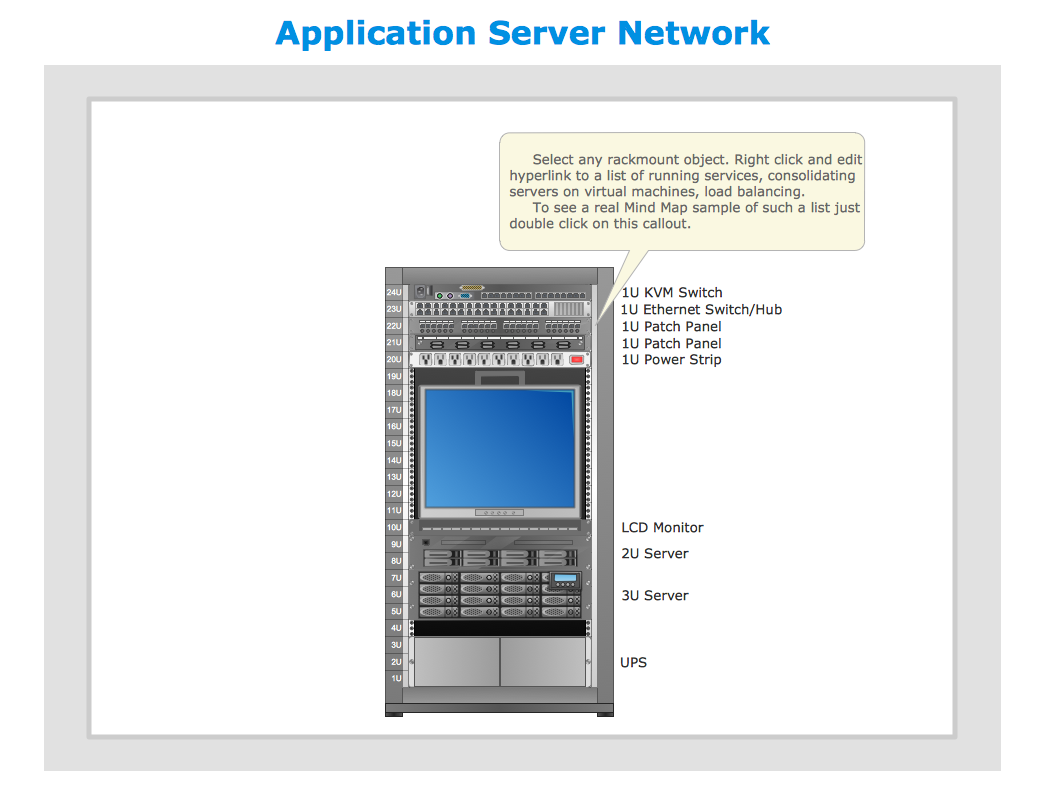## Sample 21: Home Area Networks

Network Diagram sample: : Home area networks.

This example is created using ConceptDraw DIAGRAM diagramming and vector drawing software enhanced with Computer and Networks solution from ConceptDraw Solution Park.All samples are copyrighted CS Odessa's.

Usage of them is covered by Creative Commons “Attribution Non-Commercial No Derivatives” License.

The text you can find at: https://creativecommons.org/licenses/by-nc-nd/3.0